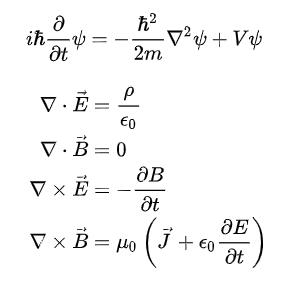# # hexo-filter-mathjax 插件使用 @Yue-plusWriting0.5.3

## # 简介

hexo-filter-mathjax 是一个用于在 Hexo 渲染 MathJax (opens new window)（数学公式）的插件。

## # 安装

npm install hexo-filter-mathjax
hexo clean


## # 配置

mathjax:
tags: none # 或 'ams' 或 'all'
single_dollars: true # 启用单个美元符号作为内联（行内）数学公式定界符
cjk_width: 0.9 # 相对 CJK 字符宽度
normal_width: 0.6 # 相对正常（等宽）宽度
append_css: true # 将 CSS 添加到每个页面
every_page: false # 如果为 true，那么无论每篇文章的前题中的 mathjax 设置如何，每页都将由 mathjax 呈现


## # 使用

---
title: On the Electrodynamics of Moving Bodies
categories: Physics
date: 1905-06-30 12:00:00
mathjax: true
---


### # 渲染器

-$\epsilon_0$
+$\epsilon_0$
-$\frac{\partial}{\partial t}$
+$\frac{\partial}{\partial t}$


-$\epsilon_0$
+$\epsilon\_0$
-\begin{eqnarray*}
+\begin{eqnarray\*}


## # 示例

$$i\hbar\frac{\partial}{\partial t}\psi=-\frac{\hbar^2}{2m}\nabla^2\psi+V\psi$$

\begin{eqnarray\*}
\nabla\cdot\vec{E}&=&\frac{\rho}{\epsilon_0}\\\\
\nabla\cdot\vec{B}&=&0\\\\
\nabla\times\vec{E}&=&-\frac{\partial B}{\partial t}\\\\
\nabla\times\vec{B}&=&\mu_0\left(\vec{J}+\epsilon_0\frac{\partial E}{\partial t}\right)\\\\
\end{eqnarray\*}